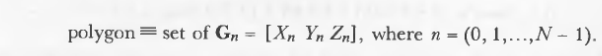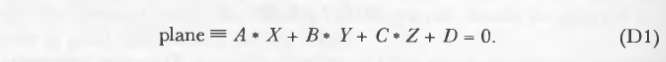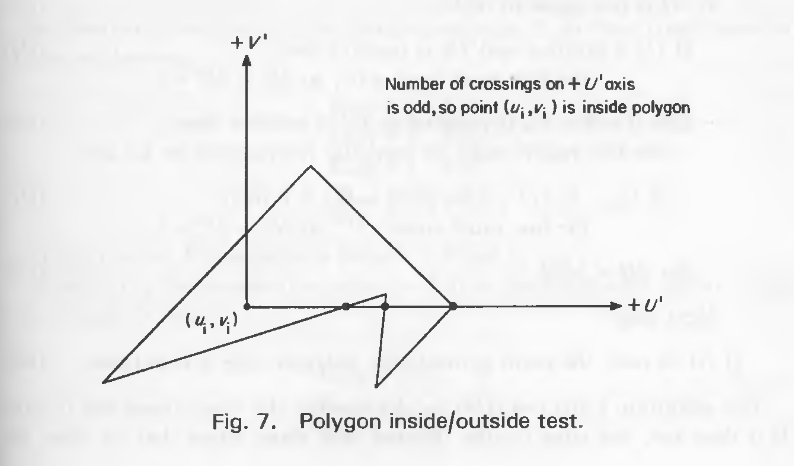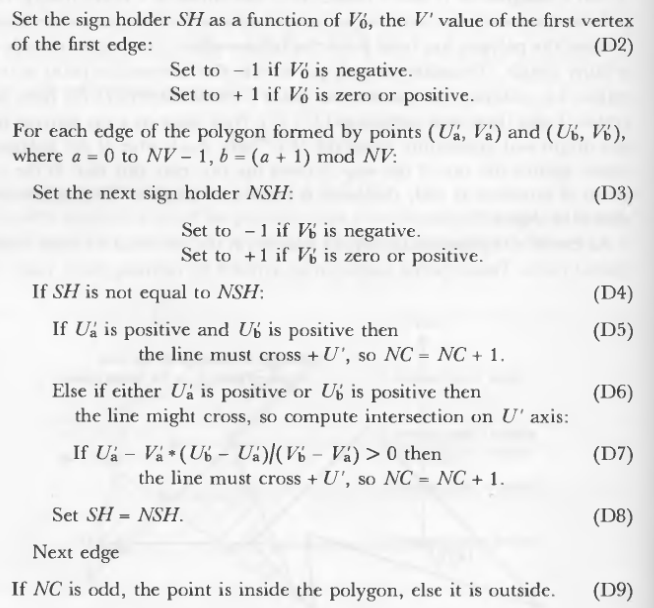• 直接求直线与多边形交点就可以过。 求的时候，先要判断线段直线是否相交，再求交点，这里的线段就是多边形的每条边。判断的话可以利用直线线段求交点的模板。我这里是利用直线的方向向量，就是题目给出的两个点...
点击打开hdu2528
这个题目看完题目后就应该知道是要求渠道与校区的交点，也就是求一条直线与多边形的交点,题目有一个重要的信息的——渠道一定会通过校园，那么题目就不用考虑特殊情况，
直接求直线与多边形交点就可以过。
求的时候，先要判断线段与直线是否相交，再求交点，这里的线段就是多边形的每条边。判断的话可以利用直线与线段求交点的模板。我这里是利用直线的方向向量，就是题目给出的两个点，这里记作向量ab,先用向量ab与多边形的每一对相邻的点求叉乘，因为相邻的两个点求出的叉乘相乘为负数，那么肯定该线段与直线相交，在求交点。
 #include<stdio.h>
#include<string.h>
#include<stdlib.h>
#include<math.h>
#include<algorithm>
using namespace std;
const double eps=1e-8;
const int maxn = 505;
struct point
{
double x,y;
};
struct ploy
{
point node[ maxn ];
int n;
};
point left,right,s;
ploy p,p1,p2;

double cross( point a,point b,point c )
{                                          ///叉乘
return (b.x-a.x)*(c.y-a.y)-(b.y-a.y)*(c.x-a.x);
}

double ploy_area( ploy res ){///求多边形面积
double ans=0;
res.node[ res.n ]=res.node;
for( int i=0;i<res.n;i++ ){
ans+=cross( s,res.node[i],res.node[i+1] );
}
return ans;
}

int dblcmp(double a) {return a<-eps?-1:a>eps?1:0;}///精度判断

ploy cut( ploy p,point s,point e )
{
point tmp;
ploy bb;
int cnt=0;
for( int i=0;i<p.n;i++ )
{
int d1,d2;
double s1,s2;
d1=dblcmp(s1=cross( p.node[i],s,e ));//跨立
d2=dblcmp(s2=cross( p.node[i+1],s,e ));//跨立
if( d1>=0 )
{
bb.node[ cnt ]=p.node[ i ];
cnt++;
}
if( d1*d2<0 )
{
tmp.x=(s2*p.node[i].x-s1*p.node[i+1].x)/(s2-s1);
tmp.y=(s2*p.node[i].y-s1*p.node[i+1].y)/(s2-s1);
bb.node[ cnt ]=tmp;
cnt++;
}
}
bb.n=cnt;
bb.node[ cnt ]=bb.node[ 0 ];
return bb;
}

int main()
{
while( scanf("%d",&p.n),p.n )
{
for( int i=0;i<p.n;i++ )
{
scanf("%lf%lf",&p.node[ i ].x,&p.node[ i ].y);
}
p.node[ p.n ]=p.node[ 0 ];

scanf("%lf%lf%lf%lf",&left.x,&left.y,&right.x,&right.y);

s.x=s.y=0;
p1=cut( p,left,right );
p2=cut( p,right,left );
int res1,res2;
res1=int(fabs(ploy_area( p1 ))/2+0.5);
res2=int(fabs(ploy_area( p2 ))/2+0.5);

printf("%d %d\n",res1>res2?res1:res2,res1>res2?res2:res1);
}
return 0;
}



展开全文• discintersect：计算两个圆盘之间的交点polydiscint：计算圆盘和多边形之间的交点plotdisc：以 3D 形式绘制光盘matlab
• -- 求多边形内点m与多边形外点n连成的线段与多边形交点且该交点坐标距离n点最近（适用任意多边形） function UIUtil:getMinPosOnPolygon(posList, inPos, outPos) -- posList:多边形顶点坐标（按照顺时针或逆...
-- 求多边形内点m与多边形外点n连成的线段与多边形的交点且该交点坐标距离n点最近（适用与任意多边形）
function UIUtil:getMinPosOnPolygon(posList, inPos, outPos) -- posList:多边形顶点坐标（按照顺时针或逆时针排序）,inPos:多边形内点的坐标，outPos:边形外点的坐标
local polygenSides = #posList -- 多边形边数
local flagPointList = {} -- 交点坐标列表

local Xm, Ym = inPos.x, inPos.y -- 线段首坐标
local Xn, Yn = outPos.x, outPos.y -- 线段尾坐标

-- 线段mn斜率 Kmn = (Yn - Ym) / (Xn - Xm)
-- 线段mn方程式 y = x * (Yn - Ym) / (Xn - Xm) + Ym - Xm * (Yn - Ym) / (Xn - Xm)
local Kmn = (Xn - Xm ~= 0) and (Yn - Ym) / (Xn - Xm) or nil
print("Kmn is ---", tostring(Kmn))

for i = 1, polygenSides do
local Xa, Ya = posList[i].x, posList[i].y -- 首坐标
local Xb, Yb = posList[i].x, posList[i].y -- 尾坐标
if posList[i + 1] then
Xb, Yb = posList[i + 1].x, posList[i + 1].y
else
Xb, Yb = posList.x, posList.y -- 最后一个点 需要与第一个点连成线段
end
local Kab = (Xb - Xa ~= 0) and (Yb - Ya) / (Xb - Xa) or nil -- 线段ab斜率
print("Kab is ---", tostring(Kab))
-- 线段ab方程式 y = x * (Yb - Ya) / (Xb - Xa) + Ya - Xa * (Yb - Ya) / (Xb - Xa)

local Xp, Yp = 0, 0 -- 交点p坐标

-- 两条线段斜率不同时 才会相交。先求出两直线交点坐标，再判断该点是否在线段ab上
if Kab and Kmn then -- 斜率都存在
if Kab ~= Kmn then -- 还要考虑斜率为0不能做分母的情况
if Kab == 0 then -- 线段ab：Yb - Ya = 0，解析式 y = Ya
Xp = (Ya - Ym + Xm * (Yn - Ym) / (Xn - Xm)) * (Xn - Xm) / (Yn - Ym)
Yp = Ya
elseif Kmn == 0 then -- 线段mn：Yn - Ym = 0，解析式 y = Ym
Xp = (Ym - Ya + Xa * (Yb - Ya) / (Xb - Xa)) * (Xb - Xa) / (Yb - Ya)
Yp = Ym
else
Xp = (Ya - Ym - Xa * (Yb - Ya) / (Xb - Xa) + Xm * (Yn - Ym) / (Xn - Xm)) / ((Yn - Ym) / (Xn - Xm) - (Yb - Ya) / (Xb - Xa))
Yp = (Xa - Xm - Ya * (Xb - Xa) / (Yb - Ya) + Ym * (Xn - Xm) / (Yn - Ym)) / ((Xn - Xm) / (Yn - Ym) - (Xb - Xa) / (Yb - Ya))
end
end
elseif Kab then --线段mn: Kmn不存在, Xn - Xm == 0, 解析式 x = Xm
Xp = Xm
if Kab == 0 then -- 线段ab：Yb - Ya = 0，解析式 y = Ya
Yp = Ya
else
Yp = Xm * (Yb - Ya) / (Xb - Xa) + Ya - Xa * (Yb - Ya) / (Xb - Xa)
end
elseif Kmn then -- 线段ab: Kab不存在, Xb - Xa == 0, 解析式 x = Xa
Xp = Xa
if Kmn == 0 then -- 线段mn：Yn - Ym = 0，解析式 y = Ym
Yp = Ym
else
Yp = Xa * (Yn - Ym) / (Xn - Xm) + Ym - Xm * (Yn - Ym) / (Xn - Xm)
end
end

print("交点坐标 is ----", table.tostring(cc.p(Xp, Yp)))
if ((Xp >= Xa and Xp <= Xb) or (Xp >= Xb and Xp <= Xa)) and ((Yp >= Ya and Yp <= Yb) or (Yp >= Yb and Yp <= Ya)) then
table.insert(flagPointList, cc.p(Xp, Yp))
end
end
print("flagPointList is ----", table.tostring(flagPointList))

-- 求所有交点中，距离n点最近的点坐标
function lenghOfTowPoint(posA, posB)
local wid = math.abs(posA.x - posB.x)
local hei = math.abs(posA.y - posB.y)
return math.sqrt(wid * wid + hei * hei)
end
local minPos -- 最近点坐标
local length -- 最近的距离
if #flagPointList > 0 then
length = lenghOfTowPoint(flagPointList, outPos)
minPos = flagPointList
for k, v in pairs(flagPointList) do
if lenghOfTowPoint(v, outPos) < length then
length = lenghOfTowPoint(v, outPos)
minPos = v
end
end
end
print("最近点的坐标 ------", table.tostring(minPos))
return minPos
end



展开全文cocos lua
• ![多边形内的平行线绘制]...##### 求一组平行线与多边形交点。如图所示，求代码思路。最好考虑凹多边形，不然只能凸包了。现在思路不清晰。求教各位了。忽略地图坐标系。在平面内计算。
• 找到两个二维多边形的边的交点，一个简单的功能，用于跟进新闻组的讨论matlab
• 计算机图形学课程多边形交点PPT 计算机科学技术一、二班
• 这一节讨论的是在平面上的一个点是否在平面上的一个多边形上。多边形被假定为完全在平面内。假设平面方程是已知的。如果不给出平面方程，就必须推导出它。参阅rogers的优秀著作，以获得正常的方法。 转载于:...
　　这一节讨论的是在平面上的一个点是否在平面上的一个多边形上。多边形被假定为完全在平面内。假设平面方程是已知的。如果不给出平面方程，就必须推导出它。参阅rogers的优秀著作，以获得正常的方法。
转载于:https://www.cnblogs.com/TooYoungTsukasa/p/9234795.html
展开全文• 一旦得到平面方程，就可以进行射线/多边形交点。在计算射线/平面交叉后，下一步是确定交点是否在多边形内。 　有许多不同的方法可以解决这个问题。Berlin很好地概述了一些技术。这里给出的方法是在中提出...
　　一旦得到平面方程，就可以进行射线/多边形的交点。在计算射线/平面交叉后，下一步是确定交点是否在多边形内。
有许多不同的方法可以解决这个问题。Berlin很好地概述了一些技术。这里给出的方法是在中提出的“射线相交”算法的一个修改版本。该算法的工作原理是，从交点处向任意方向发射一束射线，并计算相交的线段数。如果交叉的数目是奇数，则点在多边形内;其他外。这就是所谓的Jordan曲线定理。图6描述了这个定理的用法。下面提出的改进算法优雅地处理了测试光线与多边形顶点相交的特殊情况。这是我自己的发明，似乎是一个最佳的解决方案。
以点集定义定义多边形：定义平面：定义平面的法线(非必要法线)：平面与光线的交点：第一步是将多边形投射到二维平面上。在这个平面中，所有的点都由一对(U,V)指定。所以，我们想要的是每个[X Y Z]坐标都对应一个(U,V)。
一种方法是将平面绕一些轴旋转，直到法线与其他轴平行(比如Z)。在这之后，剩下的两个轴(在这个例子中是X和Y)可以用来生成(U,V)对。该方案的缺点是必须为每个多边形生成和存储一个旋转矩阵，并且必须为每个坐标执行一个矩阵乘法。
这些成本可以通过简单地丢弃[X Y Z]其中一个坐标并使用另外两个来消除。这个动作将多边形投射到由两个选定的坐标定义的平面上。多边形的面积没有保留，但是拓扑结构保持不变。选择扔掉哪个坐标的定义如下:扔掉绝对值最大的坐标。例如，对于一个Pn=[0 -5 3]的多边形，Y坐标会被丢弃，X和Z被分配给U和V (U和V是任意的)。我们将用最大的坐标作为主导坐标。
一旦多边形被投射到平面上，内外测试就相当简单了。平移多边形使交点在原点，即从每个顶点减去交点的坐标(Ui Vi)。将这些新顶点标记为(U'，V')。现在想象一条光线从原点出发沿着+U轴运动。对多边形的每条边进行相交测试。如果边缘穿过光线，记录下来。如果相交的总数是奇数，则点在多边形内。这个操作如图7所示。正如Berlin所指出的那样，在光线上的顶点必须作为特殊的情况来处理。这些特殊情况可以通过定义它们的方式来避免。沿着+U'轴延伸的光线将平面分成两部分。然而，也有一些点在U轴上。必须对位于光线上的顶点(即V' = 0)添加声明，定义其位于+ V'端。这样光线上就没有点了，特殊情况就消失了。光线本身必须被重新定义为无限接近原始光线，但不能通过任何点。它现在是一条分界线，而不是一系列的点。算法的第一次测试(D4)检查两顶点的v是否符号不同。如果符号相同，则可以忽略(因为v符号相同则不会与u交叉)。如果两U都大于0，那么+U'轴必然与其交叉(D5)。否则，如果端点中的任何一个U>0(D6)，则必须找到边与U'轴相交的准确U'位置(即此直线与U+的截距)。如果(D7)这个截距为正(即在+U'轴上)，那么这条边确实与+U'相交。
该方法比较高效，因为大多数边缘可以被平凡地拒绝或接受。 只有当边缘从对角象限延伸时才需要进行严格的计算。
这个和其他内-外部测试算法的一个小问题是，在边缘上的相交点被任意地决定在内部或外部。这个问题是有解决办法的，但是在实践中，边缘上的交点大多是不相关的。这是因为，如果一个交点落在两个多边形之间的边缘上，并且两个多边形都投影在同一个平面上，那么算法就会确定这个点在一个多边形内，并且只在其中一个多边形内(不考虑精度误差).
在图6中，五角星的中心五边形不被考虑在恒星内部，因为交叉的数量是偶数。多边形的另一个定义是将这些点考虑到多边形内部。
要对这类多边形进行内外测试，需要对之前的算法做一个简单的修改。改变的是当+U'轴的边从+V'传递到-V'时增加NC，从-V'传递到+V'时减少NC。如果NC是0，点在多边形外面，否则它在多边形里面。
数字NC被称为圈数。想象这个多边形是由绳子构成的，一个铅笔点被放在交点上。如果弦被拉紧，圈数就是弦绕点的次数。NC的符号是旋转的方向:‘+’是顺时针，‘-’是逆时针。
转载于:https://www.cnblogs.com/TooYoungTsukasa/p/9235337.html
展开全文• Are there any open source tools or libraries (ideally in python) that are available for performing lots of intersections with 3D geometry read from an ESRI shapefile? Most of the tests will be simple ...
• 多边形与多边形的交集：c++ 多边形求交集代码（凸多边形与多边形交集） #include "stdafx.h" #include <iostream> #include <stdlib.h> #include <opencv2\opencv.hpp> using namespace ...cpp
• 计算线与多边形所在面的交点 // 声明 int IntersectWithLine( double p1[ 3 ], double p2[ 3 ], double tol, double & t, double x[ 3 ], double pcoords[ 3 ], int & subId); // 测试...文档 vtk
• 提出了一种适合任意多边形裁剪的算法，该算法将构成结果多边形的裁剪多边形...简化了交点的数据结构，减少了裁剪多边形与实体多边形边界的循环求交次数．最后，通过其他同类算法进行比较，验证了算法运行的效率优势．
• 对于一个 n 个顶点的凸多边形，它的任何三条对角线都不会交于一点。请求出图形中对角线交点的个数。 例如，6 边形： 这里可以注意到并没有出现多条对角线交叉在一个点的情况。 输入格式 输入只有一行一个整数 n，...
• Compute the intersection of a line segment with a polygon. demo地址：...关键代码： // Create a square in the XY plane ...
• 主要用到IntersectWithLine（）方法，如果交叉点发生，该方法返回非0值，并且计算交叉点坐标。#include #include #include <vtkPolygon.h>int main() { vtkSmartPointer<vtkPoints> points = vtkSmartPointer...vtk
• 因为每一个交点对应两条对角线,而两条对角线又对应着一个四边形.于是焦点个数就对应四边形的个数.问题转化成由凸n边形的n个顶点取4个顶点可组成多少个四边形的问题,故最多共有n(n-1)(n-2)(n-3)/24个交点. ...
• 1.求直线段圆的交点 (1)由线段端点P1（x1,y1）P2(x2,y2)得到线段所在直线的方程    ax+by+c=0   (2)由圆心P0(x0,y0)和半径r得到圆的方程    （x-x0）2+(y-y0)2=r2   (3)由点到圆的距离公式算出圆心...c++
• 基本思想：从指定点出发，向...为了计算射线与多边形所有边的交点，我们需要遍历多边形所有的边，判断是否存在交点。这样的计算量非常大，以下给出几种可以减少计算量的方法。 一、构建安全矩形 　如图所示，构算法
• * 多边形的交，多边形的边一定是要按逆时针方向给出 * 还要判断是凸包还是凹包，调用相应的函数 * 面积并，只要和面积减去交即可 */ #include &amp;lt;bits/stdc++.h&amp;gt; using namespace std;...
• 有时需要获取直线各种图形的交点，包括多边形和各种Qt图形框。 例如上图中，要想使连接线始终在多边形的边上，且能指向多边形中心，那么我们就要获取连线AB与多边形交点。 1.多边形(QGraphicsPolygonItem)...qt
• 计算两条线或多边形交点（LineString | MultiLineString | Polygon ）Turf简介使用方法效果展示下载链接 Turf简介 Turf是用JavaScript编写的模块化GIS地理空间引擎，用于空间分析的JavaScript库。它包括传统的空间...Turf openlayers
• 取一个 Polygon|polygon 并返回 Point|points 的所有自交点。 参数 范围 类型 描述 polygon Feature.<多边形> 输入多边形 例子 var poly = { "type" : "Feature" , "properties" : { } , "geometry" : { ...JavaScript
• 描述计算两条二维线/多边形交点。 用法[X,Y]=CURVEINTERSECT(H1,H2) 或 [X,Y]=CURVEINTERSECT([H1 H2]) 或[X,Y]=CURVEINTERSECT(X1,Y1,X2,Y2) 或-> (H1,H2) 线对象句柄-> (Xi,Yi) 线的坐标-> (X,Y) 交点坐标 ...matlab...